## Result Examples - Axial Stage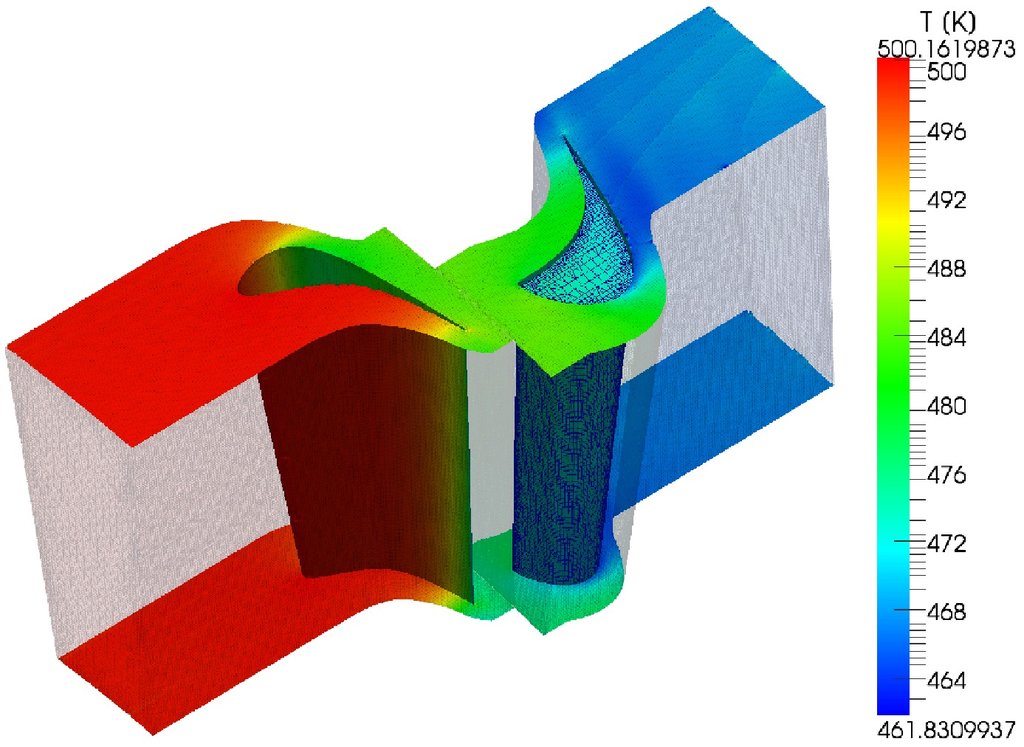Figure: Result Example – Axial Stage: Temperature distribution.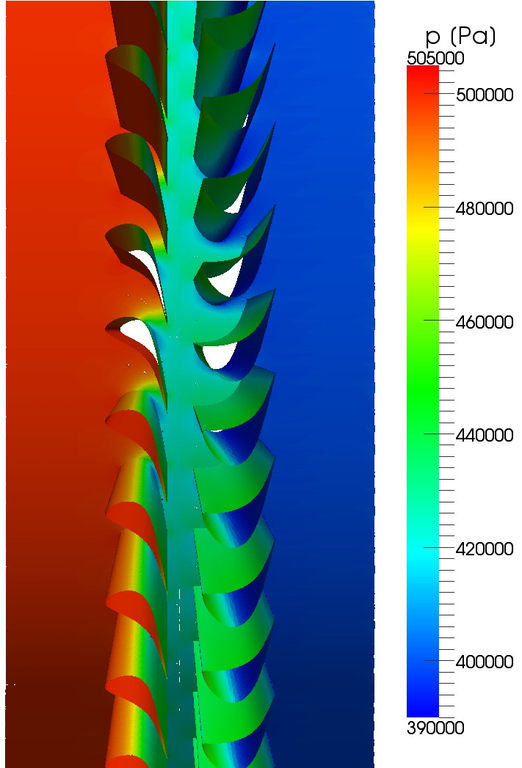Figure: Result Example – Axial Stage: Pressure distribution.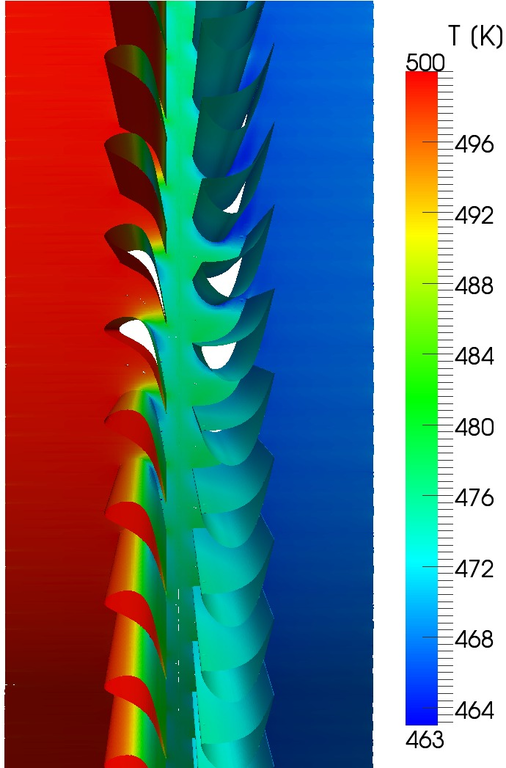Figure: Result Example – Axial Stage: Temperature distribution.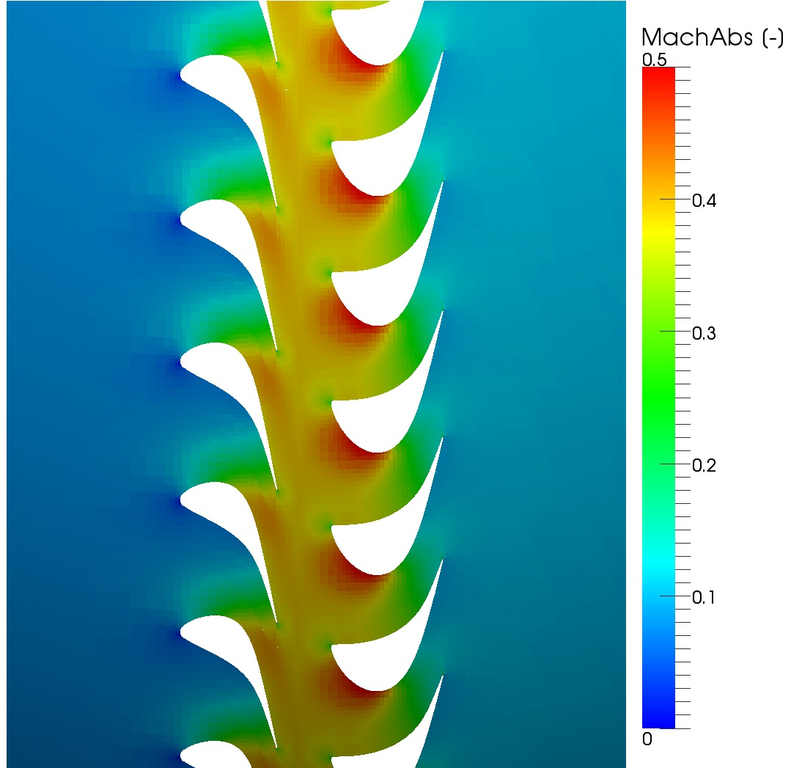Figure: Result Example – Axial Stage: Absolute Mach number.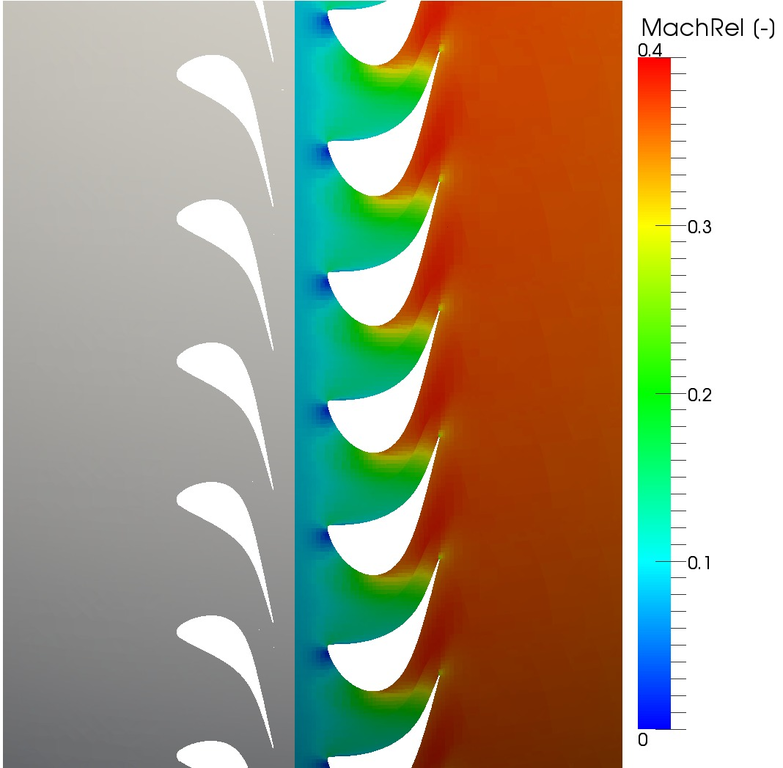Figure: Result Example – Axial Stage: Relative Mach number.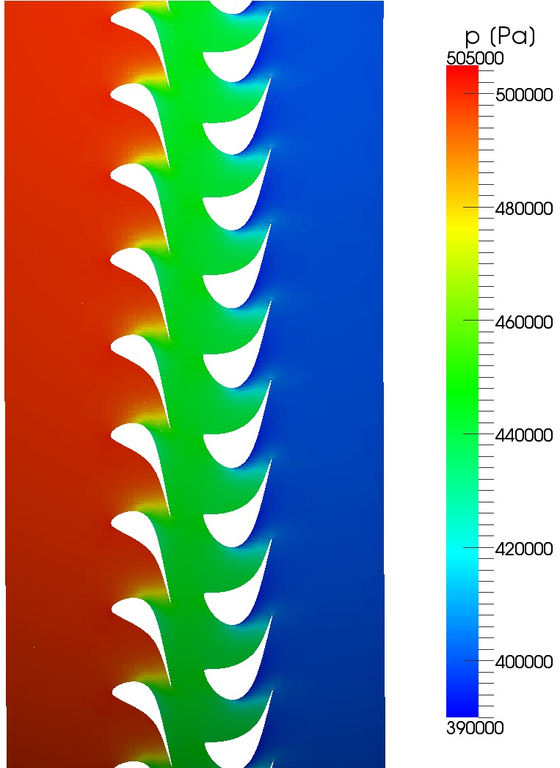Figure: Result Example – Axial Stage: Pressure distribution.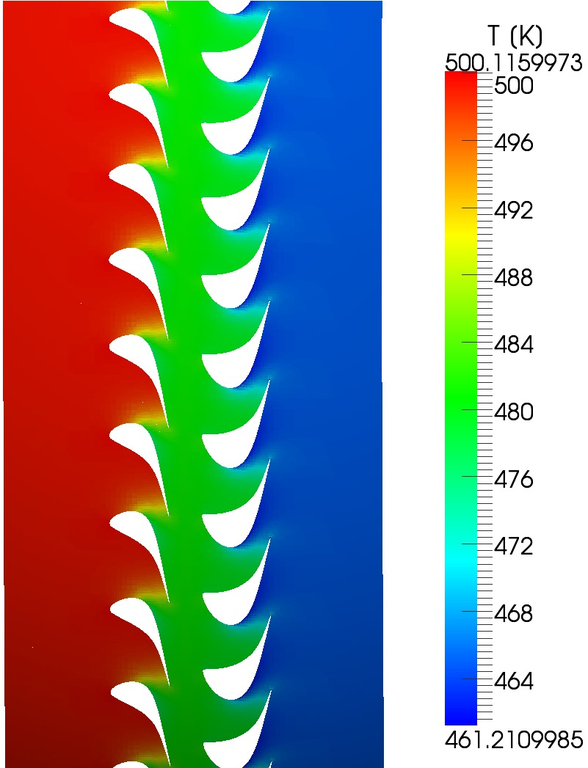Figure: Result Example – Axial Stage: Temperature distribution.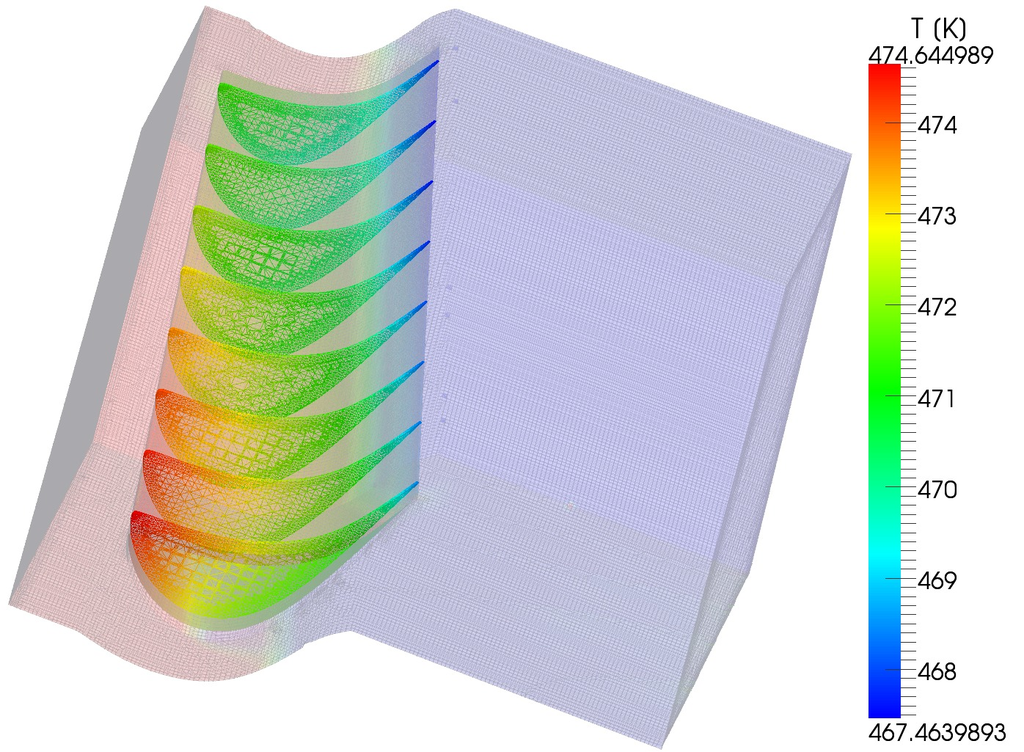Figure: Result Example – Axial Stage: Temperature distribution along the blade.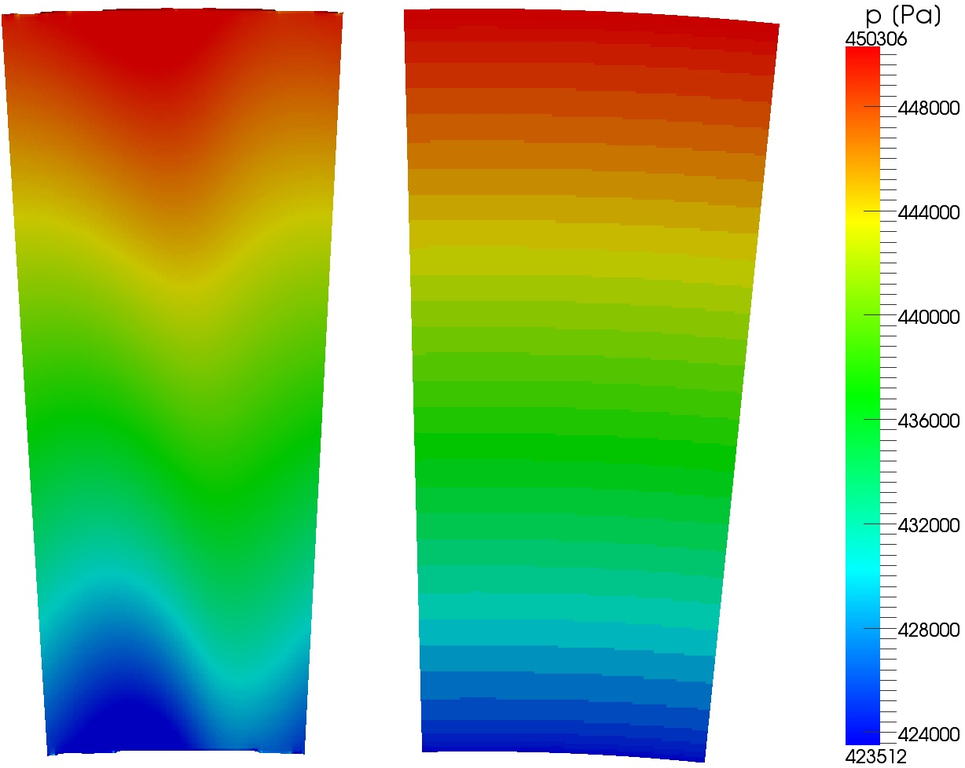Figure: Result Example – Axial Stage: Pressure distribution at the mixing plane. Rotor (left) and stator (right).